Get instant live expert help with Excel or Google Sheets“My Excelchat expert helped me in less than 20 minutes, saving me what would have been 5 hours of work!”

#### Post your problem and you’ll get Expert help in seconds.

Your message must be at least 40 characters
Our professional Expert are available now. Your privacy is guaranteed.

# How to copy data from multiple worksheets into one Excel Sheet – Excelchat

We can copy data from multiple worksheets into one by following the simple steps outlined below. In this tutorial, we will learn how to merge Excel sheets into one. We will also explore how to merge two Excel sheets, merge multiple sheets and use a VBA to combine sheets.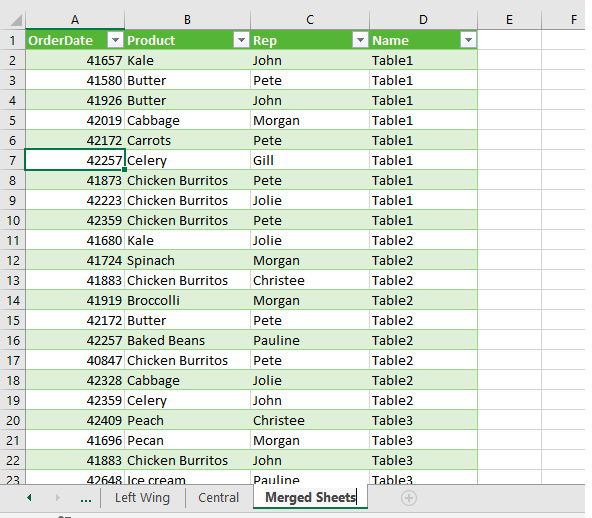Figure 1 – How to merge excel documents

## Combine Excel files into one using the Power Query tool

The Power Query tool sheets provide a quick and easy way to combine a lot of worksheets into one. When we have just a few sets of workbooks we want to merge, we can use these steps:

• We will open all the worksheets we wish to combine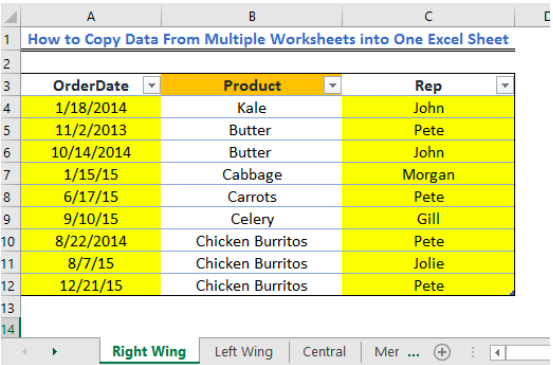Figure 2 – Sheet 1 for merge table from different sheets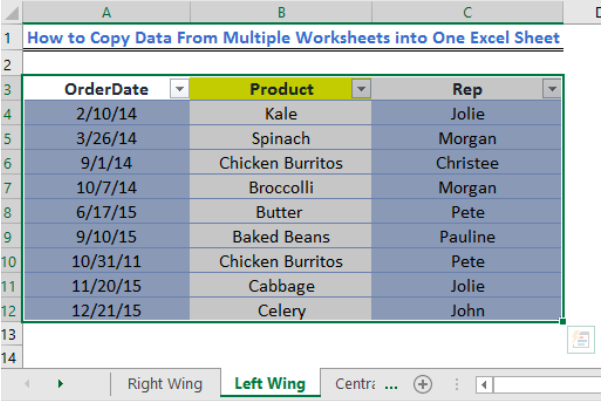Figure 3 – Sheet 2 for merging excel sheets into one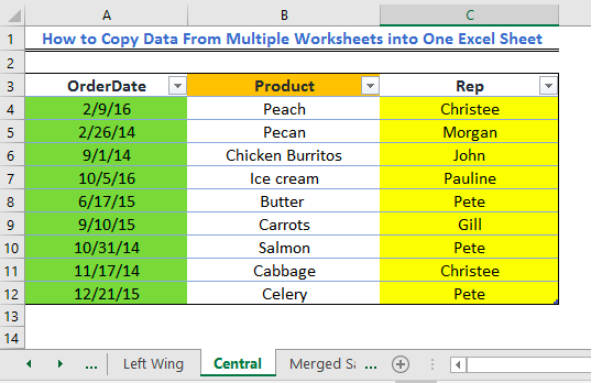Figure 4 – Sheet 3 for merging excel sheets into one

• In the blank sheet, we will go to the Data Tab
• Next, we will click on Get External Data or Get Data (depending on Excel version)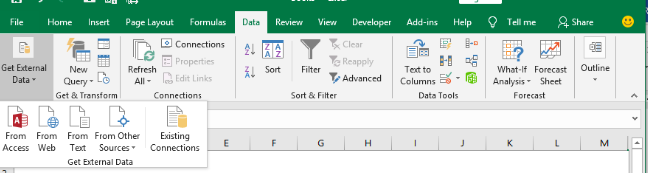Figure 5 – How to merge spreadsheets in excel

• In the drop-down list, we will go to From Other Sources option. If we have Excel 2016, we will click on New Query and select Other Sources option.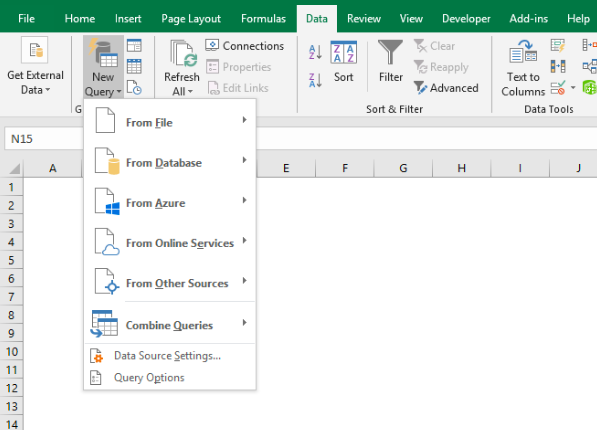Figure 6 – Merge two spreadsheets

• Next, we will click on Blank Query to open the Power Query editor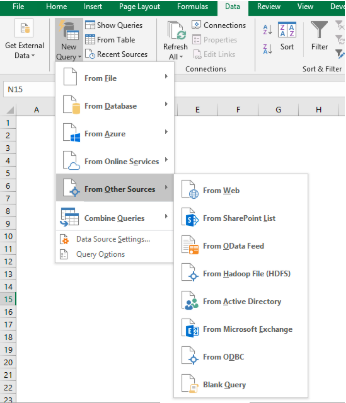Figure 7 – How to merge two Excel Sheets

• In the Editor, we will enter the formula below in the formula bar:

`=Excel.CurrentWorkbook()`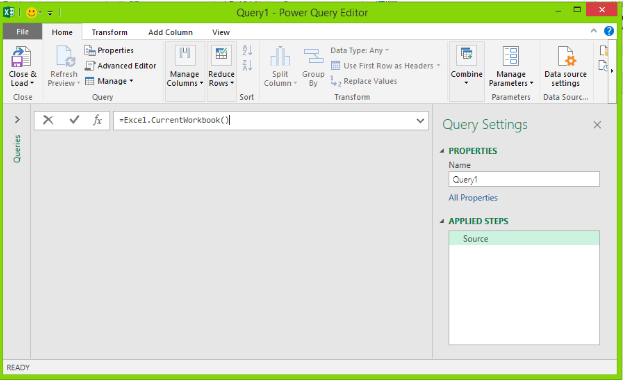Figure 8 – combine excel files

• We will hit the Enter key to show all table names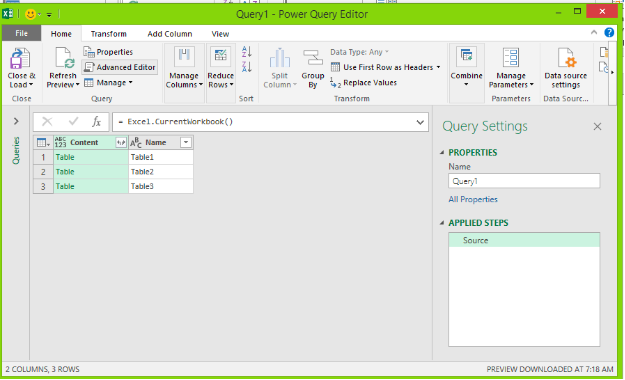Figure 9 – merge excel documents

• To combine tables, we will click on the double pointed arrow in the content header cell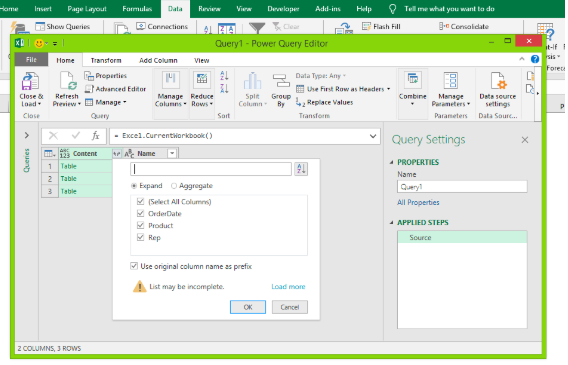Figure 10 – Merge multiple excel files

• We will select all the columns we want to combine
• We will uncheck the Use original column name as prefix option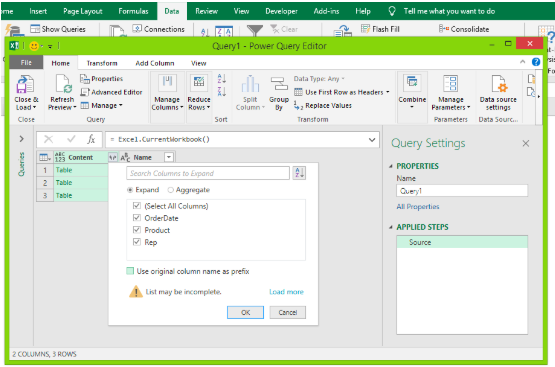Figure 11 – How to merge two Excel sheets

• We will click OK
• Our worksheets will combine into a single table in the Power Query tab.
• After combining Excel sheets in the Power Query, we can load it in Excel
• We will click on the File table
• We will click on Close and Load To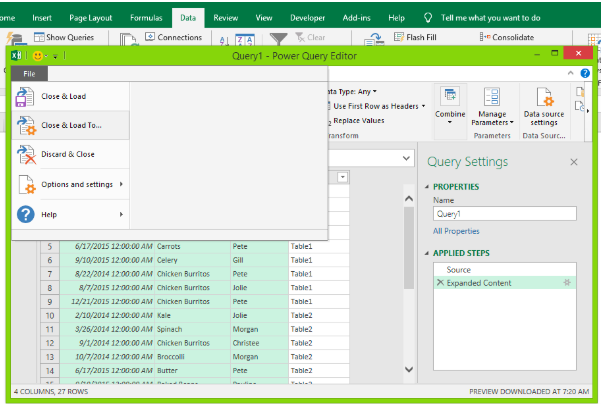Figure 12 – Merge Excel files using Power Query

• In the Import Data dialog box, we will select Table and New Worksheet option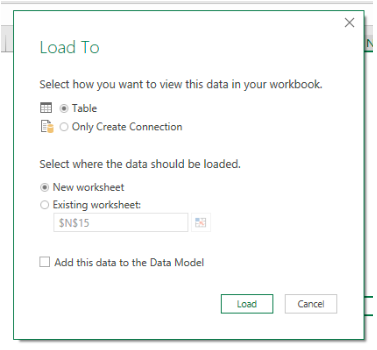Figure 13 – Combine excel files into one

• We will click OK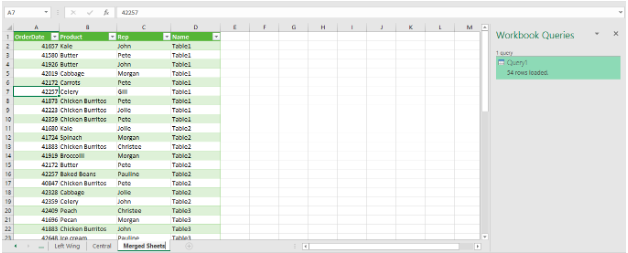Figure 14 – How to combine multiple excel files into one worksheet.

## Using a macro to combine multiple Excel files into one

When we have multiple Excel files, we can merge them in a swift manner using a VBA macro. We can easily use the macro displayed below.

• We will click on Alt + F11 and select Visual Basic Editor
• Next, we will right-click on This Workbook and select Insert, then Modules
• In the window that opens up, we will enter the code displayed below

`Sub MergeExcelFiles()`

`    Dim fnameList, fnameCurFile As Variant`

`    Dim countFiles, countSheets As Integer`

`    Dim wksCurSheet As Worksheet`

`    Dim wbkCurBook, wbkSrcBook As Workbook`

`    fnameList = Application.GetOpenFilename(FileFilter:="Microsoft Excel Workbooks (*.xls;*.xlsx;*.xlsm),*.xls;*.xlsx;*.xlsm", Title:="Choose Excel files to merge", MultiSelect:=True)`

`    If (vbBoolean <> VarType(fnameList)) Then`

`        If (UBound(fnameList) > 0) Then`

`            countFiles = 0`

`            countSheets = 0`

`            Application.ScreenUpdating = False`

`            Application.Calculation = xlCalculationManual`

`            Set wbkCurBook = ActiveWorkbook`

`            For Each fnameCurFile In fnameList`

`                countFiles = countFiles + 1`

`                Set wbkSrcBook = Workbooks.Open(Filename:=fnameCurFile)`

`                For Each wksCurSheet In wbkSrcBook.Sheets`

`                    countSheets = countSheets + 1`

`                    wksCurSheet.Copy after:=wbkCurBook.Sheets(wbkCurBook.Sheets.Count)`

`                Next`

`                wbkSrcBook.Close SaveChanges:=False`

`            Next`

`            Application.ScreenUpdating = True`

`            Application.Calculation = xlCalculationAutomatic`

`            MsgBox "Processed " & countFiles & " files" & vbCrLf & "Merged " & countSheets & " worksheets", Title:="Merge Excel files"`

`        End If`` `

`    Else`

`        MsgBox "No files selected", Title:="Merge Excel files"`

`    End If`

`End Sub`

• Now to run Macro, we will click on Alt +F8 to display Macro dialog
• Next, we will select MergeExcelFiles and tap, Run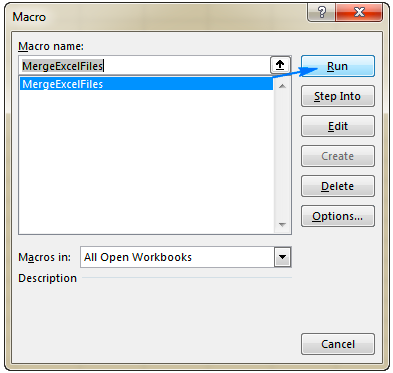Figure 15 – How to use a macro to combine excel files

## Instant Connection to an Excel Expert

Most of the time, the problem you will need to solve will be more complex than a simple application of a formula or function. If you want to save hours of research and frustration, try our live Excelchat service! Our Excel Experts are available 24/7 to answer any Excel question you may have. We guarantee a connection within 30 seconds and a customized solution within 20 minutes.